# How to use mathbb command in LaTeX?

mathbb command is used to convert uppercase and lowercase letters to blackboard-bold in terms of shape.

This command does not exist in latex by default. For this, you need to install different packages. And for different packages, there will be some structural differences between the output of this mathbb command.

If you just want to turn uppercase letters into blackboard-bold, you need to install the amsfonts, amssymb, txfonts, and fxfonts packages for the mathbb command.

 amsfonts \mathbb{ABC} →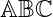amssymb \mathbb{PQR} →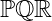txfonts \mathbb{ABC} →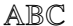pxfonts \mathbb{PQR} →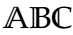\documentclass{article}
\usepackage{amsfonts}
\begin{document}
$$\mathbb{ABCDEF}$$
\end{document}

Output :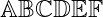You know that the sets of different types of numbering systems are written in blackboard-bold form. For example real number, natural number, complex number, … etc

\documentclass{article}
\usepackage{amsfonts}
\begin{document}
$$\mathbb{N} \; \; \mathbb{W}$$
$$\mathbb{R} \; \; \mathbb{C}$$
$$\mathbb{Z} \; \; \mathbb{Q}$$
\end{document}

Output :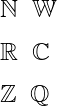And in the case of the latex program above, if you pass the lowercase letters and numbers as an argument in mathbb command, then the output will show you wrong.

Of course, you can turn lowercase letters and numbers into blackboard-bold. But, for this, you need to install the bbold package.

\documentclass{article}
\usepackage{bbold}
\begin{document}
$$\mathbb{ABC}$$
$$\mathbb{abc}$$
$$\mathbb{123}$$
$$\mathbb{ABCdef123}$$
\end{document}

Output :Exclude mathbb commands in latex. More than one command of the same type that completes the same task. For example, the varmathbb command is present in the txfonts and pxfonts packages.

\documentclass{article}
\usepackage{txfonts}
\begin{document}
$$\varmathbb{ABC}$$
$$\varmathbb{ABCdf}$$
$$\varmathbb{AaBbCc123}$$
\end{document}

Output :You can pass any expression as an argument in varmathbb command. However, varmathbb command will ignore all letters and digits except the uppercase letter.

In this tutorial, mathbb commands have been used in more than one way and have yielded more than one result. However, the best practice is to use the amsfonts and amssymb packages for mathbb commands.